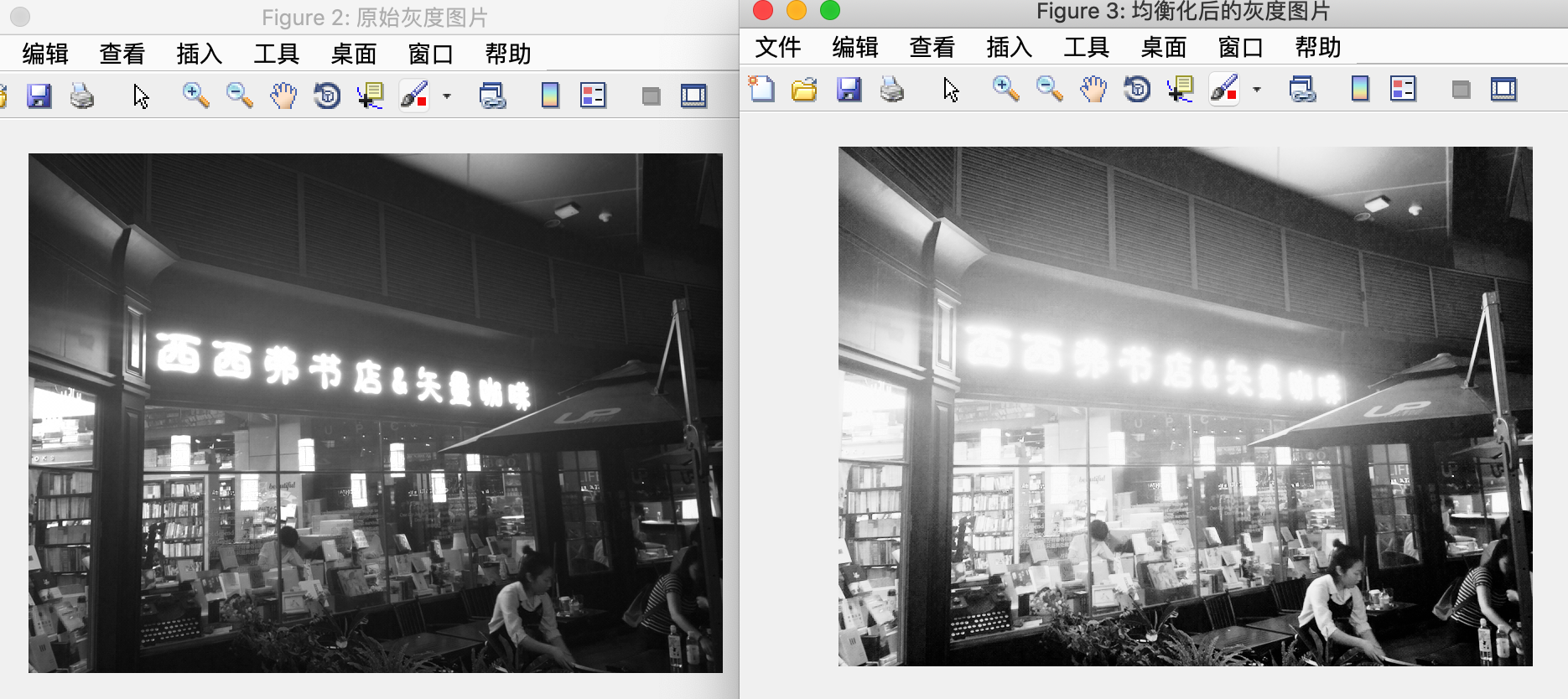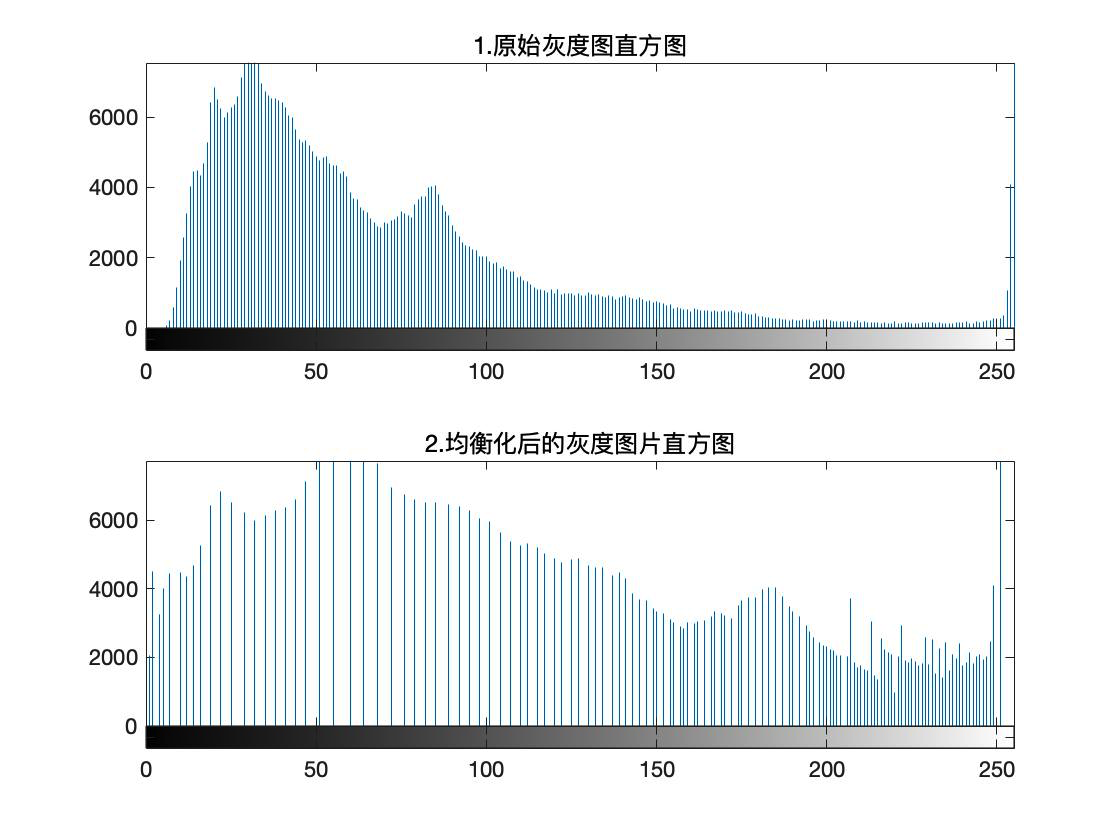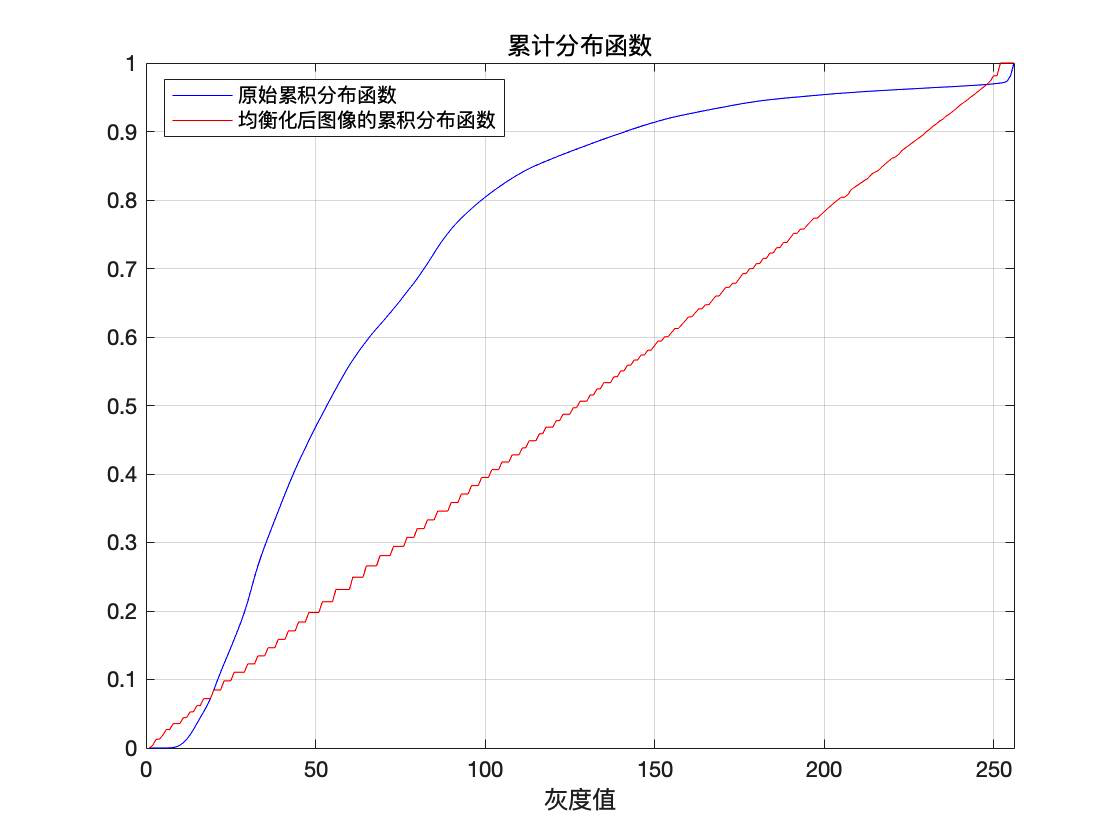• 局部直方图均衡化 Matlab
千次阅读
2017-07-08 15:14:27

步骤：1、确定模板大小 n*n

2、将图像进行扩展，因为对边界处的处理会使得图像无法与模板达到一一对应。

3、从图像的第一个像素开始，与模版点乘，点乘后的局部区域进行直方图均值化，并将局部的中心元素的作为图像的当前值

代码如下:

clear;
close all;
figure,imshow(img);
title('原始图像');

%构建模板
n=15;
model(1:n,1:n)=1;%a为n*n大小的模板，像素值为1
%对原图进行扩展，因为模板中心从图像的第一个像素开始，模板必然有点在图像外，无对应点，所以进行扩展，是的图像一一对应
imgTend=wextend('2D','sym',img,n);
tendTrans=double(imgTend);%变成double型便于后期的点乘计算
[row,col]=size(imgTend);%获取扩展后的图像的大小
tendTransTmp=tendTrans;
%model(a:b,c:d)表示A矩阵的第a到b行，第c到d列的所有元素
for i=n+1:row-n
for j=n+1:col-n %因为进行了扩展，所以原图像的第一个像素在扩展后的图像中对应的是（n+1，n+1）这个点
modelResult=tendTrans(i:i+(n-1),j:j+(n-1)).*model(1:n,1:n);%在图像中取n*n大小，与模板对应点乘，生成点乘后的n*n大小的矩阵m
modelEpual=histeq(uint8(modelResult));%对局部m进行直方图均衡化
%k=histeq(m);%对块图进行直方图均衡，m为double型，所以其k就是全1，那么x2中对应的位置也就是全1，最后显示转化成uint8显示肯定为黑色。
equalTmp=double(modelEpual);%k仍为n*n大小的矩阵
tendTransTmp(i,j)=equalTmp(8,8);%将均衡后的中心点的像素值赋给原图对应点的元素
end
end
%未被赋值的元素取原值
result=tendTransTmp(n+1:row-n,n+1:col-n);%均衡化后取原图像大小
result=uint8(result);
%d=uint8(x2);
figure,imshow(result);
title('均衡后的图像');

更多相关内容
• 局部直方图均衡化也就是对一个像素周围的区域进行直方图均衡，然后将结果返还给最中心的区域，需要自己确定模板的大小，一般都是奇数行，奇数列，运算时间较长，且模板越大，运算时间越长。 在某种意义上，像素被...

局部直方图均衡化也就是对一个像素周围的区域进行直方图均衡化，然后将结果返还给最中心的区域，需要自己确定模板的大小，一般都是奇数行，奇数列，运算时间较长，且模板越大，运算时间越长。

在某种意义上，像素被基于整幅图像的灰度分布的变换函数修改。虽然这种全局方法适用于整个图像的增强，但存在这样的情况，增强图像中小区域的细节也是需要的。这些区域中，一些像素的影响在全局变换的计算中可能被忽略了，因为全局变换没有必要保证期望的局部增强。解决方法是以图像中每个像素的邻域中的灰度分布为基础设计变换函数。
这种直方图处理技术很容易适应局部增强。该过程是定义一个邻域，并把该区域的中心从一个像素移至另一个像素。在每一个位置，计算邻域中的点的直方图，并且得到的不是直方图均衡化，就是规定化变换函数。这个函数最终用于映射邻域中心像素的灰度。然后，邻域中心的被移至一个相邻像素位置，并重复该过程。当邻域进行逐像素平移时，由于只有邻域中的一行或一列改变，所以可在每一步移动中，以新数据更新前一个位置得到的直方图。这种方法与区域每移动一个像素位置就计算邻域中所有像素的直方图相比有明显的优点。

定义参考

编写的代码如下：
程序中，选用的模板大小为11*11，且直方图程序为自己编写，也可以替换为官方函数histeq()，直方图代码思路及过程可参见直方图均衡化（自己编写函数）

注：其中的直方图均衡化函数为自己编写，也可以使用官方函数，但是效果是一样的

clc
clear;
figure(1);
imshow(I);
title('原图象'); %显示原始图象

%%
%建立模板
size_moban=11;
length = floor(size_moban/2);
a(1:size_moban,1:size_moban)=1;%模板小于图像
image=wextend('2D','sym',I,size_moban);%%扩展
%%
[M,N] = size(image);%%扩展后的图像大小
W = double(image);
result = W;

for i=1+size_moban:M-size_moban
for j=1+size_moban:N-size_moban
m=W(i:i+(size_moban-1),j:j+(size_moban-1)).*a(1:size_moban,1:size_moban); %取出x1中从(i,j)开始的n行n列元素与模板相乘
k=hist_1(uint8(m));%对局部进行直方图均衡
after=double(k);
result(i,j)=after(1+length,1+length); %将均衡后中心点的像素值赋给原图对应点的元素，未被赋值的元素取原值
end
end

Picture=result(size_moban+1:M-size_moban,size_moban+1:N-size_moban);%%均衡化后，取原图像大小
Picture=uint8(Picture);
figure;
imshow(Picture);
title('变化后的图像'); %显示均衡后的图象

%%
%直方图均衡化程序
function hist_img = hist_1(I)
[M, N] = size(I);
size_img = M*N;
c = zeros(1,256);%统计每个每个像素值的个数
b= c;%转化前后的对照表

temp = I(:);
temp = sort(temp);

for i = 1:size_img
c(temp(i)+1) = c(temp(i)+1)+1;
end
a = c;%求和
for i = 2:256
a(i) = c(i) + a(i-1);
end

min_cdf = 10000;
for i = 1:256
if a(i)>0
if a(i) < min_cdf
min_cdf = a(i);
end
end
end

for j = 1:256
if a(j) > 0
b(j) = round(255*(a(j)-min_cdf)/(size_img-min_cdf));
end
end

for i = 1:M
for j = 1:N
I(i,j) = b(I(i,j)+1);
end
end

hist_img = I;

end


效果如下：从图片中可以看出，细节部分很明显，可以通过调整模板来得到不同的效果。
参考链接：直方图均衡化（自己编写函数）

展开全文算法 计算机视觉
• 用于实现局部直方图均衡化matlab程序，需要图像处理工具箱
• 自适应直方图均衡化matlab代码从显微图像自动识别场景草多晶微藻类 乔尼·赫里贝托·吉拉尔多·祖拉加，奥古斯托·萨拉萨尔，德国迪斯，亚历山大·戈麦斯，塔蒂亚娜·马丁内斯，肯尼迪·巴尔加斯，马里亚纳·佩努埃...
• matlab直方图均衡化代码，通常用来增加许多图像的全局对比度，尤其是当图像的有用数据的对比度相当接近的时候。通过这种方法，亮度可以更好地在直方图上分布。这样就可以用于增强局部的对比度而不影响整体的对比度...
• 使用matlab实现直方图均衡化 function histgramequalization(A) %对一幅图像进行直方图均衡化 A=imread('barb.jpg'); [m,n]=size(A); B=zeros(size(A)); l=m*n; r=zeros(1,256); y=zeros(1,256); A=double(A); for i...
• 课程设计报告 题 目 基于matlab直方图均衡化程序设计 学生姓名 学生学号 系 别 专 业 届 别 指导教师 电气信息工程学院制 目 录 1 引言- 2 - 2直方图基础 - 2 - 3直方图均衡化- 3 - 3.1 直方图均衡化的概念 - 3 - ...
• 该 m 程序可用于对图像执行局部直方图均衡化。 如果需要，请参考内置函数 roipoly 和 histeq。
• 摘要：本文主要讨论了直方图均衡化处理的图像增强技术的基本原理，并用Matlab语言实现了直方图均衡化的图像增强处理。实验结果表明，直方图均衡化处理能有效地改善图像的对比度，改善图像的灰度层次。关键词：图像...

摘要：本文主要讨论了直方图均衡化处理的图像增强技术的基本原理，并用Matlab语言实现了直方图均衡化的图像增强处理。实验结果表明，直方图均衡化处理能有效地改善图像的对比度，改善图像的灰度层次。

关键词：图像增强 灰度级 直方图均衡化 Matlab

中图分类号：TP751 文献标识码：A 文章编号：1007-9416(2012)01-0174-02

枷裨銮考际跏鞘�滞枷翊�硌芯康幕�径韵笾�弧Ｔ銮康闹饕�康氖鞘雇枷癜刺囟ǖ男枰�怀鐾枷裰械哪承┬畔���保�魅趸虺鋈ツ承┎恍枰�男畔�1]。图像空间域增强技术是数字图像增强的一个重要应用，是以对图像像素的直接处理为基础，通过线性或非线性变换来增强构成图像像素的一种技术。本文所讨论的直方图均衡化增强方法便属于这种方法。

1、直方图处理

灰度级直方图是灰度级的函数，是描述一幅图像中灰度级与出现这种灰度的概率之间的关系的图形，是图像最基本得统计特性。直方图是多种空间域处理技术的基础，直方图操作能有效地用于图像增强。

为了便于数字图像处理，图像的直方图须引入离散形式。灰度级为[0,L-1]范围的数字图像的直方图的离散函数为：

h(rk)=nk (1)

其中rk是第k级灰度，nk是图像中灰度级rk的像素个数。在图像中，像素的灰度级要作归一化处理一遍计算机进行处理，用图像中像素的总数n来除它的每个值，得到归一化直方图:

环�枷竦幕叶燃�被归一化到区间[0,1]，且r=0代表黑色，r=1代表白色。对于一幅给定的图像，每一个像素取得[0,1]区间内的灰度级是随机的，那么图像灰度级r可被看作为区间[0,1]的随机变量，就可以用概率密度函数pr(r)来表示原始图像的灰度分布。令s为增强后的图像灰度级像素值，相应可以用概率密度函数ps(s)来表示增强后的图像灰度分布。

可以对[0,1]区间内原始图像的任意一个灰度级r值进行如下变换，得到输出灰度级s：

s=T(r) (3)

通过上述变换，每个原始图像的灰度值r都对应产生一个增强后图像的灰度级s值。

变换函数T(r)满足两个条件：(1)T(r)在区间0≤r≤1中为单值且单调递增；(2)当0≤r≤1时，0≤T(r)≤1。条件(1)中单值是为了保证反变换的存在，单调条件保持图像从黑到白的顺序；条件(2)保证输出灰度级与输入灰度级有同样的范围。从s到r的反变换函数可以表示为：

r=T-1(s) 0≤s≤1 (4)

由基本概率理论可知：如果pr(r)和T(r)已知，且T-1(s)满足条件(1),则有：

因此，直方图技术的基础就是通过变换函数T(r)控制输入图像灰度级的概率密度函数，改变输出图像的灰度层次，从而达到增强图像的目的。

2、直方图均衡化

直方图均衡化处理是以累积分布函数变换法为基础的。对于连续图像，变换函数为：

由此可见，用r的累积分布函数作为变换函数可产生一幅灰度级分布具有均匀概率密度函数的图像，该图像的灰度级较为均匀化，且覆盖了整个范围[0,1]。灰度级均衡化的最终处理结果是扩展了图像像素取值的动态范围，具有较高的对比度。

3、Matlab实现

利用Matlab实现直方图均衡化技术对图像进行处理函数格式如下：

(1)h=imhist(f,b)

(2)g=histeq(f,n)

说明：对于格式(1)，显示图像f的直方图，b为用于形成直方图的灰度级的个数，默认值为256；格式(2)对图像f进行均衡化处理，n为输出图像指定的灰度级数，默认值为64。

举例说明直方图均衡化处理：

figure,imshow(f); %显示图像

title("原始图像")

figure,imhist(f) %原始图像直方图

title("原始图像直方图")

g=histeq(f,256); %均衡化处理

figure,imshow(g);

title("均衡化处理结果")

figure,imhist(g); %均衡化后的直方图

title("均衡化后的直方图")

程序实现的图像为图(a)和(b),从中可以看出，原图像(a)的灰度级动态范围窄而集中于灰度级的中部，导致了图像的对比度低而使整幅图像模糊不清。经过直方图均衡化处理后，直方图的成分覆盖了灰度级很宽的范围，而且像素的分布没有太不均匀，处理后的图像(b)变的清晰了，图像中的许多细节被突出了。

4、结语

直方图是多种图像空间域处理技术的基础，能有效地用于图像增强，直方图均衡化是图像增强的基本技术。本文分析了直方图均衡化处理方法的基本理论，并用Matlab进行了实验。结果表明，直方图均衡化方法并不能生成平坦的直方图，但它具有能增强图像灰度级的动态范围的特性。

参考文献

(美)冈萨雷斯等著，阮秋琦等译.数字图像处理[M].2版.北京：电子工业出版社，2007:70―81.

阮秋琦.数字图像处理学[M].北京：电子工业出版社,2001:181―195.

(美)冈萨雷斯等著,阮秋琦等译.数字图像处理(Matlab版)[M].北京:电子工业出版社，2005:54―61

李耀辉,刘保军.基于直方图的图像增强[J].华北科技学院学报,2003,5(2):65-66.

汪志云,黄梦为等.基于直方图的图像增强及其Matlab实现[J].计算机工程与科学，2006,28(2):54―56.

本文为全文原貌 未安装PDF浏览器用户请先下载安装 原版全文

展开全文• 此处为方便理解，不使用MATLAB中自带的histeq，手写代码...完整MATLAB代码如下： clc clear %% 1.图片预处理 L = 256; % 划分的灰度级,一般也默认为256 img = imread('西西弗.jpg'); % 读图 img_gray = img(:,:,1)...

此处为方便理解，不使用MATLAB中自带的histeq，手写代码了解内在原理。

原始彩色图片如下：均衡化后的图像如下：从累计分布函数的图像可以明显看出，均衡化只需要用一次即可，第二次几乎没有作用，因为均衡化的累计分布函数已经是一条斜率为1的函数，表明其函数已经服从均匀分布~！
完整MATLAB代码如下：

clc
clear

%% 1.图片预处理
L = 256;                    % 划分的灰度级,一般也默认为256
img_gray = img(:,:,1);             % 将3通道彩色图片转换为1通道灰度图像

%% 2.计算每个灰度级的概率分布P(离散值)和累计分布S
N = imhist(img_gray);         % 直方图函数默认划分256个灰度级
for i = 1:size(N)
P(i) = N(i)/sum(N);
if i == 1
S(1) = P(1);
else
S(i) = S(i-1) + P(i);
end
end

%% 3.将累计分布S映射回R,得到均衡化后的R
r = round((L-1).*S+0.5);

%% 4.根据均衡化后的R,生成均衡化后的灰度图像
img_equal = img_gray;
for i = 1:size(img_equal(:))
value = img_equal(i);
if value == 0            % 灰度值value∈[0,255],但matlab中索引从1开始
img_equal(i) = 1;
else
img_equal(i) = r(value);
end
end

%% 5.绘图
figure('NumberTitle', 'on', 'Name', '原始彩色图片'),imshow(img)
figure('NumberTitle', 'on', 'Name', '原始灰度图片'),imshow(img_gray)
figure('NumberTitle', 'on', 'Name', '均衡化后的灰度图片'),imshow(img_equal)

figure
subplot(2,1,1);imhist(img_gray,L),title('1.原始灰度图直方图');   % 划分为L个灰度级
subplot(2,1,2);imhist(img_equal,L),title('2.均衡化后的灰度图片直方图');   % 划分为L个灰度级

figure('NumberTitle', 'on', 'Name', '累积分布函数'),plot(1:256,S)
axis([0 256 0 1]) % X象限∈[0,255],Y∈[0,1]

展开全文图像处理 图像增强
• 直方图均衡化Matlab实现 步骤： 统计直方图 累计直方图 映射 close all clear all clc srcImage = imread('lena.jpg'); srcImage = rgb2gray(srcImage); grayImage = srcImage; [height,width] = size(grayImage)...图像处理 算法
• 数字图像处理课程的第三次作业，包括图像灰度直方图的绘制、基于直方图的各种空域处理。参考课本是冈萨雷斯的《数字图像处理》
• 直方图均衡化 简介 直方图是图像中像素强度分布的图形表达方式。它统计了每一个强度值所具有的像素个数。直方图均衡化是通过拉伸像素强度分布范围来增强图像对比度的一种方法。是图像处理领域中利用图像直方图对...计算机视觉 算法
• 全局直方图均衡处理和局部直方图均衡化处理 算法思想 计算直方图函数，申请一个全零长度为256的向量，存储对应减一的像素值个数。通过遍历原图的每一个像素值，将遍历到的像素值在对应的向量中加1，最后按照向量中的...图像处理
• 理解和掌握直方图、直方图均衡、直方图匹配的原理和实现方法；自己编程实现均衡matlab 函数的结果进行比较；采用直方图匹配重新处理图像，匹配...采用局部直方图均衡对图像进行处理，以获得黑方块内的图像信息。
• 空域增强就是直接对图像的像素进行处理，主要有灰度变换、直方图方法等，直方图均衡化就是改善图像质量非常好的一种方法；频域增强可以先将图像由空间域转换到频域后，在频域进行滤波，频域滤波可以用到低频滤波、...图像处理
• 直方图均衡化是基于灰度直方图的图像增强的一种方法，还有另外一种方法是直方图规定。 均衡的目的是将原始图像的直方图变为均衡分布的的形式，将一非均匀灰度概率密度分布图像，通过寻求某种灰度变换，变成一幅...
• matlab开发-直方图均衡和局部直方图均衡。图像的直方图均衡和局部直方图均衡
• 直方图均衡化对图像进行非线性拉伸，重新分配图像像元值，使一定灰度范围内像元值的数量大致相等就是直方图的均衡。原来直方图中间的峰顶部分对比度得到增强，而两侧的谷底部分对比度降低，输出图像的直方图是一个...
• 一、图像增强及直方图均衡化简介 1 图像增强 图像增强是对图像的某些特征，如边缘、轮廓、对比度等进行强调或锐化，以便于显示、观察或进一步分析与处理。通过对图像的特定加工，将被处理的图像转化为对具体应用来说...图像处理 计算机视觉
• 直方图均衡化 定义 当直方图中像素值集中在狭窄的灰度级范围内或分布极不均匀时，图像呈现较差的对比度。直方图均衡化的目的就是将直方图的灰度级概率分布变换为均匀分布。(如下图所。注意，p(x)是概率密度函数，P...
• 基于matlab的图像处理，直方图均衡化,图像直方图均衡化局部均衡，可使图像灰度级均衡平坦
• 这样就可以用于增强局部的对比度而不影响整体的对比度，直方图均衡化通过有效地扩展常用的亮度来实现这种功能。 这种方法对于背景和前景都太亮或者太暗的图像非常有用，这种方法尤其是可以带来X光图像中更好的骨骼.....图像识别 计算机视觉
• ## matlab实现直方图均衡化

万次阅读 多人点赞 2018-04-16 15:34:34
直方图均衡化是一种利用灰度变换自动调节图像对比度质量的方法，基本思想是通过灰度级的概率密度函数求出灰度变换函数，它是一种以累计分布函数变换法为基础的直方图修正法。直方图均衡化处理的步骤如下：1. 求出...
• 文章目录main.mcompute_cdf.m实验图像效果 main.m clear; clc; rgb = imread('girl.jpg');...title('原'); % 划分4*4的网格，分别计算cdf [m, n] = size(gray); cdf = zeros(16, 256); for i=1:4 for j=1:4计算机视觉
• 直方图均衡化、直方图变换、对比度自适应直方图均衡化
• Matlab代码引入了双边直方图均衡化（BHE），这是一种基于融合的策略，用于实现局部自适应色调映射，该映射在由联合双边滤波器的扩散功能隐式定义的支持上近似直方图均衡化。 我们强烈建议阅读相关论文用于X...
• 实现彩色图像的直方图均衡化算法 实验过程 算法流程可以参考灰度图像的直方图均衡化算法 （传送门） 测试代码 F=imread('.\im\f11.jpg'); [G] = imhisteq_C(F); figure,imshow(F);figure,imshow(G); 实验所需图片 ...算法 opencv 计算机视觉 机器学习...

# 局部直方图均衡化matlab代码matlab 订阅